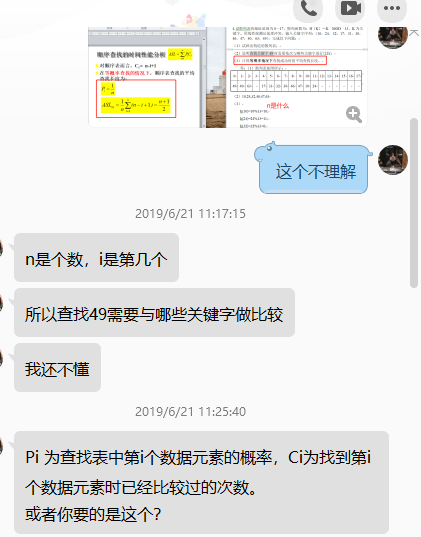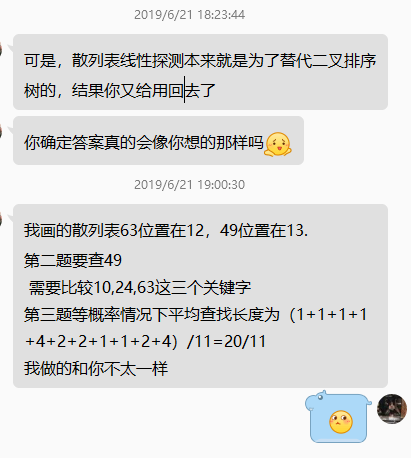# 等概率情况下查找成功时的平均查找长度

4.设散列表的地址范围为0～17，散列函数为：H（K）＝K MOD 13，K为关键字。用线性探测法处理冲突，输入关键字序列：（10，24，32，17，31，30，46，47，40，63，49），完成以下问题：
（1）试画出构造的散列表；
（2）说明查找关键字49时需要依次与哪些关键字进行比较；
（3）计算等概率情况下查找成功时的平均查找长度。

0 1 2 3 4 5 6 7 8 9 10 11 12 13 14 15 16 17
49 40 63 17 31 32 30 46 47 10 24
（2）10,24,32,46,47,63
（3）19/9
h(10)=10%13=10;
h(24)=24%13=11;
h(32)=32%13=6;
h(17)=17%13=4;
h(31)=31%13=5;
h(30)=30%13=4;(冲突)
h0=4;
h1=(4+1)%11=5; (冲突)
h2=(5+1)%11=6; (冲突)
h3=(6+1)%11=7;
h(46)=46%13=7;(冲突)
h0=7;
h1=(7+1)%11=8’
h(47)=47%13=8;(冲突)
h0=8;
h1=(8+1)%11=9;
h(40)=40%13=1;
h(63)=63%13=11; (冲突)
h0=11;
h1=(11+1)%11=1; (冲突)
h2=(1+1)%11=2
h(49)=49%13=10; (冲突)
h0=10;
h1=(10+1)%11=0;09-176万+04-189万+
10-302410
12-161932
04-218831
12-282000
04-221万+
11-111万+
11-281512
10-221008
09-161万+
04-201290
02-148584
09-023487
09-227060
03-3183
05-061万+
05-061万+